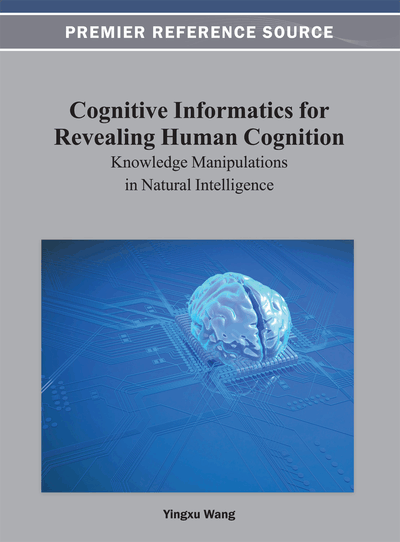# Equivalence between LDA/QR and Direct LDA

Rong-Hua Li (The Hong Kong Polytechnic University, Hong Kong), Shuang Liang (The Hong Kong Polytechnic University, Hong Kong), George Baciu (The Hong Kong Polytechnic University, Hong Kong) and Eddie Chan (The Hong Kong Polytechnic University, Hong Kong)
DOI: 10.4018/978-1-4666-2476-4.ch021
Available
\$37.50
No Current Special Offers

## Abstract

Singularity problems of scatter matrices in Linear Discriminant Analysis (LDA) are challenging and have obtained attention during the last decade. Linear Discriminant Analysis via QR decomposition (LDA/QR) and Direct Linear Discriminant analysis (DLDA) are two popular algorithms to solve the singularity problem. This paper establishes the equivalent relationship between LDA/QR and DLDA. They can be regarded as special cases of pseudo-inverse LDA. Similar to LDA/QR algorithm, DLDA can also be considered as a two-stage LDA method. Interestingly, the first stage of DLDA can act as a dimension reduction algorithm. The experiment compares LDA/QR and DLDA algorithms in terms of classification accuracy, computational complexity on several benchmark datasets and compares their first stages. The results confirm the established equivalent relationship and verify their capabilities in dimension reduction.
Chapter Preview
Top

## 1. Introduction

Classical Linear Discriminant Analysis (LDA) algorithm usually suffers from the singularity problem (Ye et al., 2004; Chen et al., 2000) of scatter matrices. Linear Discrimiant Analysis via QR decomposition (LDA/QR) (Ye & Qi, 2000; Ye & Li, 2004) and Direct Linear Discriminant Analysis (DLDA) (Yu & Yang, 2005) are two LDA algorithms to solve this singularity problem. This paper proves the equivalence relationship between these two LDA algorithms.

LDA/QR is a LDA extension for coping with the singularity problem and was developed in (Ye & Qi, 2000). It improves the efficiency by introducing QR decomposition on a small-sized matrix, while achieving competitive discriminant performance. There are two stages-of-process in LDA/QR: (1) In the first stage, the separation between different classes is maximized via applying QR decomposition to a small-sized matrix. Remind that this stage can be used independently as a dimension reduction algorithm and it is called Pre-LDA/QR (Ye & Li, 2004); (2) The second stage refines the result by applying LDA to the so-called reduced scatter matrices (Ye & Qi, 2000) resulting from the first stage. The solution by LDA/QR has been proved to be equivalent to that by generalized LDA named pseudo-inverse LDA (Ye et al., 2004; Zhang et al., 2005).

DLDA (Yu & Yang, 2005) bases on the observation that the null space of between-class scatter matrix carries little useful discriminative information (Chen et al., 2000). Accordingly, it first diagonalizes between-class scatter matrixand then adopts traditional eigen-analysis to discard the zero eigenvalues together with their corresponding eigenvectors. Then, it diagonalizes within-class scatter matrixbut keeps the zero eigenvalues since its null space carries the most useful discriminant information. Hence DLDA can make use of all discriminant information. One advantage of DLDA is that it can be applied to high-dimensional input space directly without any intermediate dimension reduction stage, such as PCA (Martinez & Kak 2001; Turk & Pentland, 1996; Yang & Yang, 2006), which has been used in the preprocessing stage of a well-known face recognition algorithm, namely fisherfaces (Belhumeur et al., 1997; Jing et al., 2006).

## Complete Chapter List

Search this Book:
Reset